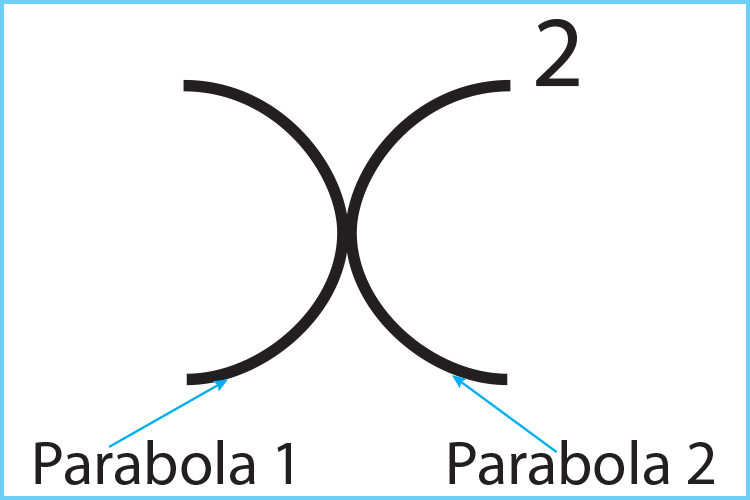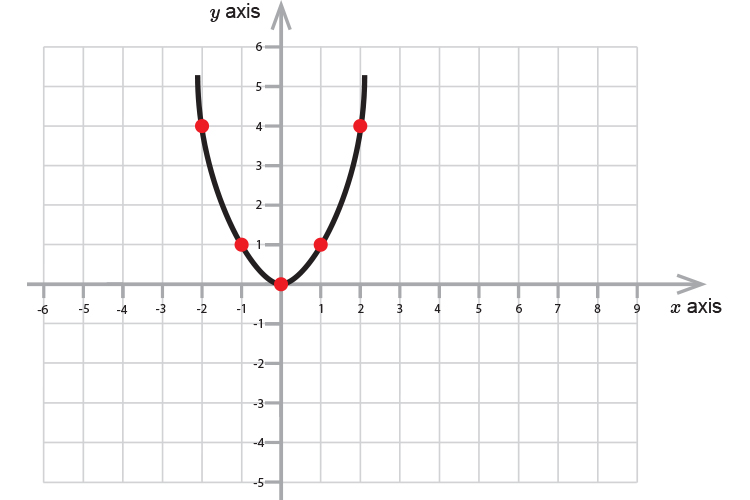# The most famous parabola

The most famous parabola is:

y=x^2

To remember this thinkThere are 2 parabolas in x^2

And it's very easy to work out what y=x^2 is if you plot figures of x against y.

x=2
Therefore
y=2^2=4
x=1
Therefore
y=1^2=1
x=0
Therefore
y=0^2=0
x=-1
Therefore
y=-1^2=1
x=-2
Therefore
y=-2^2=4

Which can be plotted as